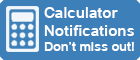Calculator Library
Free Online CalculatorsHome » 87 Calculators » Business » Depreciation Calculator

Huge Domain Name Sale | Free Domains
Domain names on sale at crazy prices. Register a new domain for FREE when you buy another service.

## DEPRECIATION CALCULATOR

INSTRUCTIONS

This calculator is designed to work out the depreciation of an asset over a specified number of years using either the Straight Line or Reducing Balance Methods.

Straight Line Method Depreciation Calculator

Historical Cost of Goods
Number of years to depreciate over

Annual Depreciation Amount
Monthly Depreciation Amount
Depreciation Period
Reducing Balance Method Depreciation Calculator

Original Cost of Goods
Annual Depreciation Rate %

Year 1 Depreciation Amount
Year 1 Written Down Value
Year 2 Depreciation Amount
Year 2 Written Down Value
Year 3 Depreciation Amount
Year 3 Written Down Value
Year 4 Depreciation Amount
Year 4 Written Down Value
Year 5 Depreciation Amount
Year 5 Written Down Value